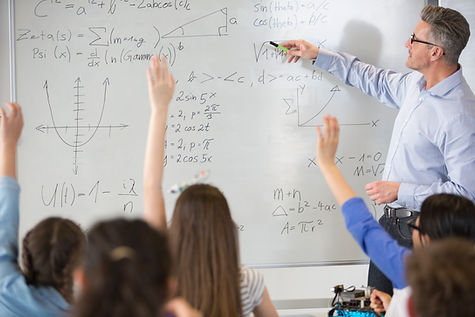top of page

# ENGINEERING ANALYSIS ILEARNING TOOLS SITE

Ordinary Differential Equations and Linear Algebra## ALGEBRA AND CALCULUS PRE-REQUISITES

At the beginning of the course, you are required to take an exam on the following topics: algebraic manipulation, partial fraction decomposition, differentiation, and integration.## FIRST-ORDER ORDINARY DIFFERENTIAL EQUATIONS

This chapter covers fundamentals of differential equations, and solution methods for 1st-order ODEs, such as separation of variables, the linear method, and exact equations. Furthermore, you'll learn about real-life applications of 1st-order ODEs, such as the growth/decay equation, and Newton's law of cooling.## SECOND-ORDER DIFFERENTIAL EQUATIONS

This chapter covers the solution of linear 2nd-order ODEs. Involves solution of homogeneous equations with constant coefficients and undetermined coefficients. Moreover, we'll study the application of these equations to spring-mass systems.## THE LAPLACE TRANSFORM

Laplace transforms are a powerful tool for solving different types of ODEs. Try to memorize the formulas to speed up your solution process.## INTRODUCTION TO LINEAR ALGEBRA

We will learn different linear algebra topics applied in multiple engineering courses, such as solution of linear systems of algebraic equations, computation of eigenvalues and eigenvectors, and solution of linear systems of ODEs by diagonalization.

Home: Tests & Assignments
bottom of page